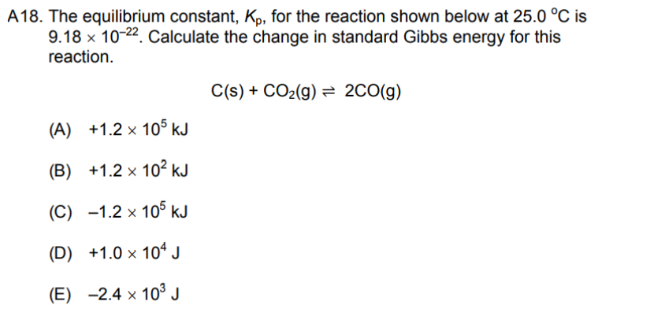# Problem: The equilibrium constant, Kp, for the reaction shown below at 25.0°C is 9.18 x 10-22. Calculate the change in standard Gibbs energy for this reaction. C(s) + CO2(g) ⇌ 2CO(g) a. +1.2 x 105 kJ b. +1.2 x 102 kJ c. -1.2 x 105 kJ d. +1.0 x 104 J e. -2.4 x 103 J

###### FREE Expert Solution
85% (267 ratings)###### Problem Details

The equilibrium constant, Kp, for the reaction shown below at 25.0°C is 9.18 x 10-22. Calculate the change in standard Gibbs energy for this reaction.

C(s) + CO2(g) ⇌ 2CO(g)

a. +1.2 x 105 kJ

b. +1.2 x 102 kJ

c. -1.2 x 105 kJ

d. +1.0 x 104

e. -2.4 x 103 J